# Quantum Mechanical Model

## Quantum Mechanical Model

It is the theoretical science that deals with the study of motion of microscopic objects that have both particle and wave nature.
Quantum mechanics is based on a fundamental equation which is called Schrodinger equation.
Schrodinger’s equation: For a system (such as an atom or a molecule whose energy does not    change with time) the Schrödinger equation is written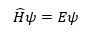Here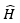is a mathematical operator called Hamiltonian.
Schrödinger gave a recipe of constructing this operator from the expression for the total energy of the system. The total energy of the system takes into account the kinetic energies of all the sub- atomic particles (electrons, nuclei), attractive potential between the electrons and nuclei and repulsive potential among the electrons and nuclei individually. Solution of this equation gives E and ψ.
Features of Quantum Mechanical Model
• The energy of electrons in an atom is quantized i.e. hour certain fixed values.
• The existence of quantized electronic energy level is direct reset of wave properties of electron.
• Both exact position and exact velocity of electron cannot be determined simultaneously.
• IMPORTANT:- An atomic orbital has wave function ψ There are many orbital’s in an atom. Electrons occupy an orbital which has definite energy. An orbital can have more than two electrons the orbital are filled in increasing order of energy. All the information about an electron is stored in orbital wave function
• The probability of finding an electron in a point within an atom is proportional to ψ2 it is known as probability density and it is always positive from the value of ψ2 at different points. It is possible to predict the region around the nucleus where the electrons are most probability found.
Atomic orbital can be specified by giving their corresponding energies & angular momentum which are quantaised & expressed in terms of quantum numbers.

## Quantum Numbers

There are a set of four quantum numbers which specify the energy, size, shape and orientation of an orbital. To specify an orbital only three quantum numbers are required while to specify an electron all four quantum numbers are required.

Four quantum number are
n, l, m, s

### Principle quantum number (n):

1. This quantum number tells energy level or shell to which electron belong
n = 1, 2, 3,….
2. This also gives information about – average distance of electron from nucleus.
• Energy of e- in hydrogen & hydrogen like atoms.
1. Max no. of electron present in a shell is given by 2n2
2. Number of orbital in a shell is given by n2
 n 1 2 3 4 No of Orbitals(n2) 1 4 9 16 Max number of electron (2n2) 2 8 18 32

### Azimuthal/ subsidiary/ Orbital angular quantum number(l)-

1. This gives us information about:
1. No. of sub – shell
2. angular momentum of electron
3. Shapes of various orbitals
4. energy of orbitals in multi-electron atoms along with principal quantum number
The number of orbitals in a subshell = 2l + 1. For a given value of n, l can have n values ranging from 0 to n-1. Total number of subshells in a particular shell is equal to the value of n.

 Subshell s p d f g Value of ‘l’ 0 1 2 3 4 Number of orbitals 1 3 5 7 9

Subshell Notation
 n l Subshell notation 1 0 1s 2 0 2s 2 1 2p 3 0 3s 3 1 3p 3 2 3d 4 0 4s 4 1 4p 4 2 4d 4 3 4f

### Magnetic Quantum number (ml )

It gives information about the No. of preferred orientations of electrons in sub – shell. Each orientation corresponds to orbital
Therefore   ml gives no. of orbitals in a sub shell
For any sub-shell (defined by ‘l’ value) 2l+1 values of ml are possible.
 L ml 0 0 (1 s orbital) 1 -1,0,1 (3 p orbital) 2 -2, -1,0,1,2 ( 5 d orbital) 3 -3,-2,-1,0,1,2,3 ( 7 f orbital) 4 -4,-3,-2,-1,0,1,2,3,4  (9 g orbitals)

### Electron Spin quantum number (ms )

It refers to orientation of the spin of the electron. It can have two values +1/2 and -1/2. +1/2 identifies the clockwise spin and -1/2 identifies the anti- clockwise spin.

## SHAPES OF ORBITAL

Probability Density
ψ gives us the amplitude of wave. The value of ψ has no physical significance.
|Ψ|2 gives us the region in which the probability of finding an electron is maximum. It is called probability density.

### Nodal surfaces

The region where this probability density function reduces to zero is called nodal surfaces or simply nodes.

Radial nodes occur when the probability density of wave function for the electron is zero on a spherical surface of a radius. Number of radial nodes = n – l – 1

### Angular nodes

Angular nodes occur when the probability density wave function for the electron is zero along the directions specified by a particular angle. Number of angular nodes = l
Total number of nodes = n – 1

### Boundary Surface Diagrams or Orbitals

It is surface in the space where probability density is constant for a given orbital. This gives  a good representation of the shape of the orbital. This shapes encloses the volume or region where probability of finding electron is high

‘S’ orbital’s                      All the s -orbital are Spherical shape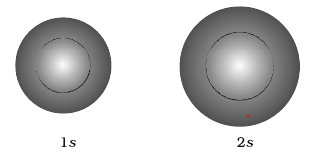Shape of P orbital – It has 3 possible orientation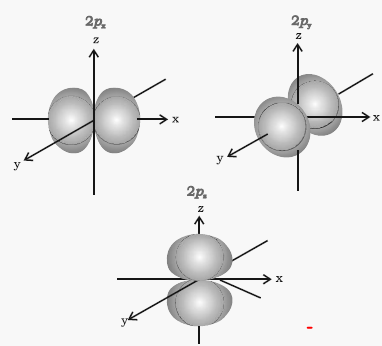Shape of ‘D’ Orbital
It has five orientations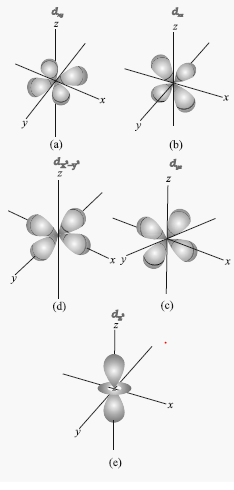## Energy in the Orbital

### Degenerate orbitals

Orbitals having the same energy are called degenerate orbitals.

### Shielding effect or screening effect

Due to the presence of electrons in the inner shells, the electron in the outer shell will not experience the full positive charge on the nucleus.
So, due to the screening effect, the net positive charge experienced by the electron from the nucleus is lowered and is known as effective nuclear charge. Effective nuclear charge experienced by the orbital decreases with increase of azimuthal quantum number (l).
The energy of the orbital is determined by sum of the principle quantum number and Azimuthal quantum number. (n + l)

## Rules for the filling of electrons in the Orbital’s

### Aufbau rule or Principle

It states that in ground state of atom, the orbitals are filled in increasing order of their energies.
The sum of the values of the principal quantum numbers (n) and azimuthal quantum number (l) i.e (n+l) determined the energy level of an orbital.
The lower value of the sum of (n+l) implies that the energy of the orbital is low. If the value of n+l for two orbitals is equal then the orbital with a lower value of n will have a lower energy level.
As per this rule ,the order in which electrons are filled
1s, 2s ,2p, 3s ,3p ,4s ,3d ,4p, 5s,4d,5p,4f,5d,6p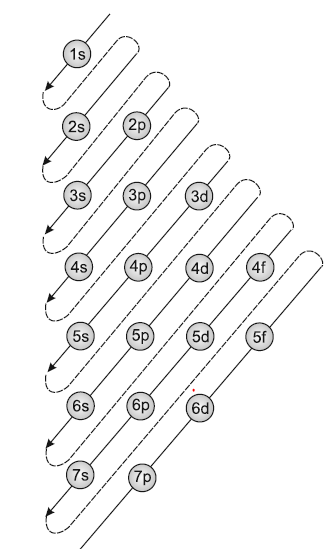### Pauli exclusion Principle

According to this principle no two electrons can have same set of 4 quantum no.
It can be also stated as only two electron can exists in the orbital  and these electrons must have opposite spin
So s subshell can have maximum 2 electrons
p -subshell can have maximum 6 electrons
d- subshell can have maximum 10 electrons

### Hund’s rule of maximum multiplicity

It states that pairing of electrons in the orbital’s belonging to same sub – shell [ p, d, f], does not take place until each orbital gets singly occupied.
All the orbitals which are singly occupied have parallel spins which can be either clockwise or anticlockwise.
Electrons have parallel spin have less inter-electronic repulsions and high spin multiplicity

## Electronic configuration of atoms

Electronic configuration is the Arrangement of electrons in different orbitals of an atom. The electronic configuration of different atoms can be represented in two ways.
a. sa pb dc...... notation.
b. Orbital diagram: each orbital of the subshell is represented by a box and the electron is represented by an arrow (↑) a positive spin or an arrow (↓) a negative spin
Few important note
1) the electron in the  completely filled shell are called core electrons
2) The electrons that are added to the electronic shell with the highest principle quantum number is called Valence electrons

## Why Electronic configuration of atoms is required

The knowledge of Electronic configuration of atoms is required to help us understand the chemical properties of the various elements

## Stability of completely filled and half filled subshells:

Symmetrical distribution of electrons- the completely filled or half-filled sub-shells have  symmetrical distribution of electrons in them and are more stable.

Exchange energy-The two or more electrons with the same spin present in the degenerate orbitals of a sub-shell can exchange their position and the energy released due to this exchange is called exchange energy. The number of exchanges is maximum when the subshell is either half filled or completely filled. As a result the exchange energy is maximum and so is the stability.
Question 1
Write the electronic Configuration for the following atomic numbers
a) 23
b) 30
c) 19
d) 38
e) 25
Solution
We know the rules of filling the electron in the orbit are
a) As per Aufbau rule or Principle,the orbitals are filled in increasing order of their energies.
1s, 2s ,2p, 3s ,3p ,4s ,3d ,4p, 5s,4d,5p,4f,5d,6p
b) There can be maximum two electrons in the orbital
c) pairing of electrons in the orbital’s belonging to same sub – shell [ p, d, f], does not take place until each orbital gets singly occupied
So p subshell can be have maximum 6 electrons and d-subshell have 10 electrons
So electronic configuration can be written as
a) 1s2 , 2s2, 2p6 ,3s2 ,3p6,4s2 ,3d3
b) 1s2 , 2s2, 2p6 ,3s2 ,3p6,4s2 ,3d10
c) 1s2 , 2s2, 2p6 ,3s2 ,3p6,4s1
d) 1s2 , 2s2, 2p6 ,3s2 ,3p6,4s2 ,3d10 ,5s2 ,4p6
e) 1s2 , 2s2, 2p6 ,3s2 ,3p6,4s2 ,3d5
Question 2
a) How many subshell are possible with n = 4
b) How many e- will be present in subshell having ms = -1/2 for n = 4.
Solution
For n = 4
l = 0 to (n – 1)
= 0, 1, 2, 3
l = 0      ml = -l to + 1 =0
l = 1      ml = -l to + l =-1, 0, 1
l = 2      ml = -l to + l = -2, -1, 0, 1, 2
l=3        ml = -l to + l = -3, -2, -1, 0, 1, 2, 3
For ms =-1/2 there will be 16 electrons
Question 3
Give the no. of unpaired electrons in Iron (Fe26)
Solution
Electronic Configuration of Fe26 = 1s2 ,2s2,2p6 ,3s2, 3p6 ,4s2,3d6
So, there will be ‘4’ unpaired electrons.
Question 4
Give the no. of unpaired e- in Fe +3
Solution
Electronic Configuration of Fe26 = 1s2 ,2s2,2p6 ,3s2, 3p6 ,4s2,3d6
Now For Fe+3
E. C. is: 1s2 2s2 2p6 3p2 3p6 3d5
Question 5
(i) Write E. C. of 19th e- in chromium (24)
(ii) Give all 4 values of quantum no. for 19th e-.
Solution
Predicted E. C. of chromium: -
=1s2 2s2 2p6 3s2 3p6 4s2 3d4
Actual  E. C. of chromium: - = 1s2 2s2 2p6 3s2 3p6 4s1 3d5
The reason is half filled subshell are more stable      So, configuration of 19th electrons:
4s1   n=4,l=0,ml =0, ms =1/2

Question 6
Which of the following set of quantum no. is impossible?
a) n = 3, l = 2, m = -2, s = +1/2
b) n = 4, l = 0, m = 0, s = +1/2
c) n = 3, l = 2, m = -3, s = +1/2
d) n = 5, l = 3, m = 0, s = +1/2
Solution
n = 3
l = 0 to (n – 1)
= 0 to  2
0, 1, 2
s, p, d
m = -l to + l
l = 0      ml = -l to + 1 =0
l = 1      ml = -l to + l =-1, 0, 1
l = 2      ml = -l to + l = -2, -1, 0, 1, 2